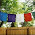## Sunday, February 21, 2010

### Two EAMCET (Medical) 2009 Questions on Photons and Electrons

Today we will discuss two multiple choice questions from modern physics. These questions are from the section ‘electrons and photons’ and they were included in the EAMCET (Medical) 2009 question paper:

(1) In Millikan’s oil drop experiment, a charged oil drop of mass 3.2×10–14 kg is held stationary between two parallel plates 6 mm apart, by applying a potential difference of 1200 V between them. How many electrons does the oil drop carry?

(1) 7

(2) 8

(3) 9

(4) 10

The electric field ‘E’ between the plates is V/d where V is the potential difference and d is the separation between the plates.

Therefore E = 1200/(6×10–3) = 2×105 volt per metre.

The electric force on the drop is Eq where ‘q’ is the charge on the drop. Since the drop is stationary, the electric force balances the weight mg of the drop.

Therefore Eq = mg so that

q = mg/E = (3.2×10–14×10)/( 2×105) = 1.6×10–18 coulomb.

Since the electronic charge is 1.6×10–19 coulomb, the number of excess electrons carried by the drop is (1.6×10–18) /(1.6×10–19) = 10.

(2) Electrons accelerated by a potential of ‘V’ volts strike a target material to produce ‘continuous X-rays’. Ratio between the de Broglie wave length of the electrons striking the target and the shortest wave length of the ‘continuous X-rays’ emitted is

(1) h/√(2Vem)

(2) (1/c) √(2m/Ve)

(3) (1/c) √(Ve/2m)

(4) he/√(Ve/2m)

The de Broglie wave length λ of the electron is given by

λ = h/p where h is Planck’s constant and p is the momentum of the electron.

The shortest wave length λmin of the ‘continuous X-rays’ produced by the striking electrons is given by

hc/λmin = Ve where c is the speed of light in free space and e is the electronic charge.

[Note that we have equated the entire energy of the electron to the energy of the X-ray photon for obtaining minimum wave length (or, the maximum frequency νmax):

eV =max = hc/λmin].

Therefore, λmin = hc/Ve.

The ratio of wave lengths required in the problem is

λ /λmin = (h/p)/(hc/Ve ) = Ve/pc………..(i)

The kinetic energy of the electron is eV and the momentum p of the electron is related to its kinetic energy as

eV = p2/2m where m is the mass of the electron.

Therefore, p = √(2mVe)

Substituting this in Eq (i), we obtain

λ /λmin = (1/c) √(Ve/2m)

#### 1 comment:

1.i like the thomas edison quote widget on the bottom of the page!